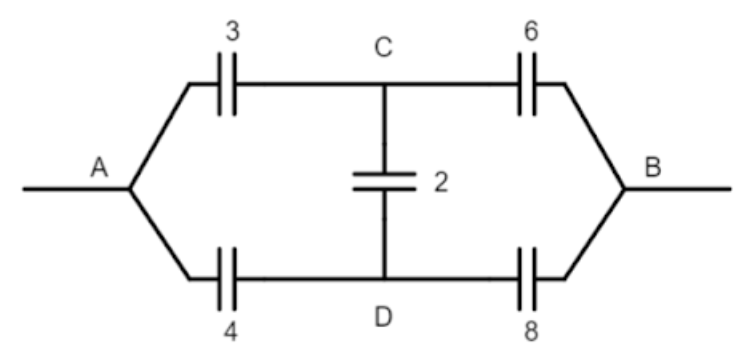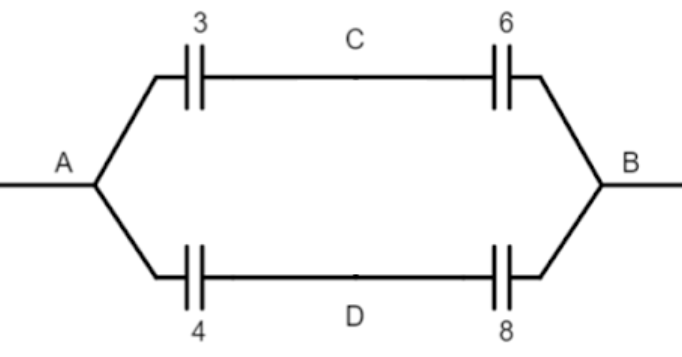QuestionAnswers

# Effective capacitance between A and B in the figures shown is (all capacitances are in μF)A. $\dfrac{3}{14}$μFB. $\dfrac{14}{3}$μFC. 21 μFD. 23 μFVerified
129k+ views
Hint: We will find the electric potential at points A, B, C, and D using the formula, and then we will find the charges stored on each of the capacitors. The relation of the charge stored on the capacitors along the same line will be the same and we will use this to find the effective capacitance.

Formula used:
The relation between capacitance-voltage across the capacitor and charge stored in the capacitor
$Q=CV$.

Complete step-by-step solution:
Let ${{Q}_{2}},{{Q}_{3}},{{Q}_{4}},{{Q}_{6}},{{Q}_{8}}$ be the charge stored on the capacitors of 2 μF, 3 μF, 4 μF, 6 μF and 8 μF respectively. Then their values will be
\begin{align} & {{Q}_{2}}=2({{V}_{D}}-{{V}_{C}}) \\ & {{Q}_{3}}=3({{V}_{A}}-{{V}_{C}}) \\ & {{Q}_{4}}=4({{V}_{A}}-{{V}_{D}}) \\ & {{Q}_{6}}=6({{V}_{C}}-{{V}_{B}}) \\ & {{Q}_{8}}=8({{V}_{D}}-{{V}_{B}}) \\ \end{align}
As the capacitors of 3 μF and 6 μF lie on the same line they will have the same charge on them. Similarly, for the capacitors of 4 μF and 8 μF.
\begin{align} & {{Q}_{3}}={{Q}_{6}}\Rightarrow 3({{V}_{A}}-{{V}_{C}})=6({{V}_{C}}-{{V}_{B}})\Rightarrow ({{V}_{A}}-{{V}_{C}})=2({{V}_{C}}-{{V}_{B}})\Rightarrow 3{{V}_{C}}={{V}_{A}}+2{{V}_{B}} \\ & {{Q}_{4}}={{Q}_{8}}\Rightarrow 4({{V}_{A}}-{{V}_{D}})=8({{V}_{D}}-{{V}_{B}})\Rightarrow ({{V}_{A}}-{{V}_{D}})=2({{V}_{D}}-{{V}_{B}})\Rightarrow 3{{V}_{D}}={{V}_{A}}+2{{V}_{B}} \\ \end{align}
As we can see above, the potentials as points C and D are the same which means that no current will flow between them and through the 2 μF capacitor. So, it will be like there is no line connecting the points C and D. So now the capacitors of 3 μF and 6 μF will be in series on the same branch which will be parallel to another branch which capacitors of 4 μF and 8 μF will be in series as in the figure.The capacitance of the branches in series will be$\dfrac{1}{\dfrac{1}{3}+\dfrac{1}{6}}=2$ μF and $\dfrac{1}{\dfrac{1}{4}+\dfrac{1}{8}}=\dfrac{8}{3}$ μF. These two will be in parallel to each other so the effective capacitance will be $\dfrac{8}{3}+2=\dfrac{14}{3}$ μF. Hence, the correct option will be B, i.e. $\dfrac{14}{3}$μF.

Note: Take care when solving this question. Although the potential at points C and D will be the same, there won’t be any current flowing between them. There can be a common mistake of considering that the points C and D will be connected to each other. That way we will get the same answer, but that is not what actually happens.